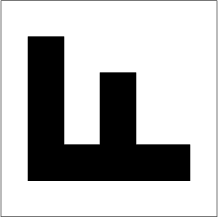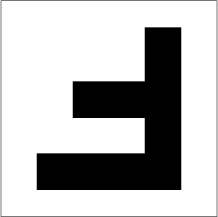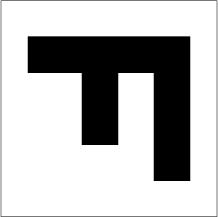# D4 Explorer

The group of symmetries of a square, known as D4, is generated by two operations: counter-clockwise rotation by a quarter turn (denoted a) and reflection across a horizontal axis (denoted b).

Clicking each button performs the indicated operation. The aggregate transformation is shown in “standard form” above each square. The equation below the diagrams indicates the algebraic operation that transforms the left-hand diagram into the right. (Remember that function composition is read from right to left. For example, the product ab is the transformation obtained by reflecting first, then rotating.)